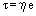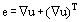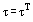# NEWTONIAN FLUIDS

A Newtonian fluid is one where there is a linear relationship between stress and strain-rate. The ratio of stress to strain-rate is the Viscosity of the fluid. A fluid where there is no linear relationship between stress and strain-rate is called a Non-Newtonian fluid.
For a Newtonian fluid the stress (strictly, the deviatoric stress) τ is given by:
(1)where e denotes the strain-rate given in terms of the velocity u by:
(2)and η denotes the viscosity of the fluid, which is independent of e, though it may depend on temperature T and perhaps on pressure p.
Because e is a symmetrical tensor, it follows from (1) that t too is a symmetrical tensor:
(3)Angular momentum conservation then implies that Newtonian fluids are nonpolar and hence, that the angular momentum is just the moment of the linear momentum.
Most fluids under normal circumstances are Newtonian so the study of Newtonian fluids comprises the major part of the subject of fluid mechanics. Linear momentum conservation then leads to the Navier-Stokes equations.